2021-2022 GCSE Mathematics Foundation Grades 1-5
Introduction To Angles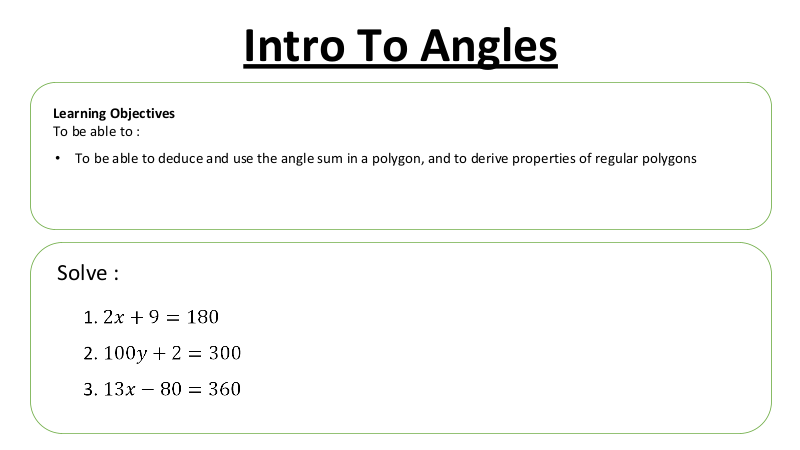Angles In Polygons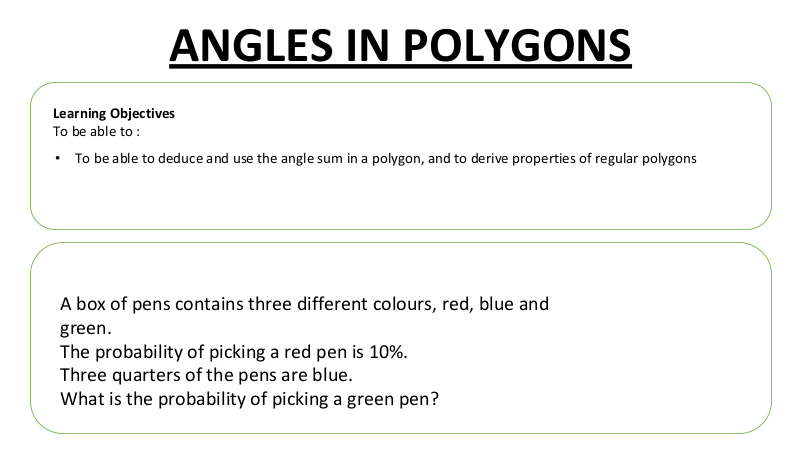Angles and Shapes PREP SHEET WEEK 9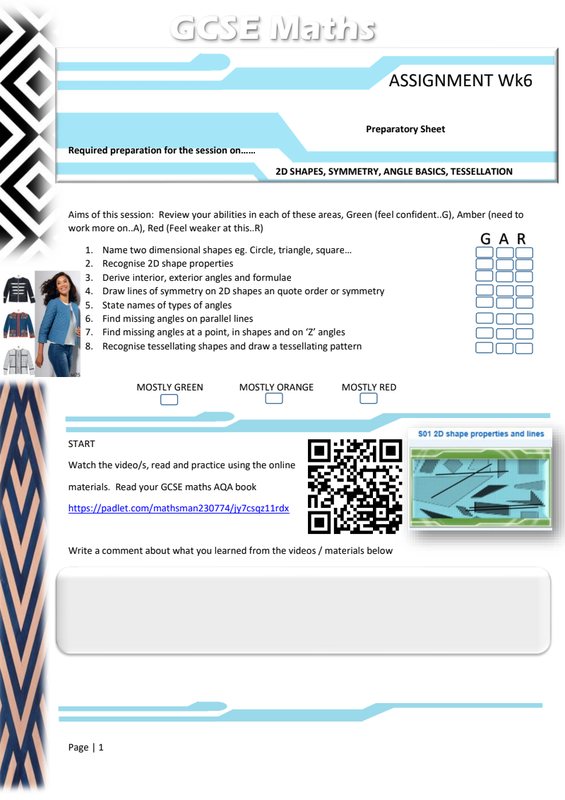Lesson Visual Summary Sheet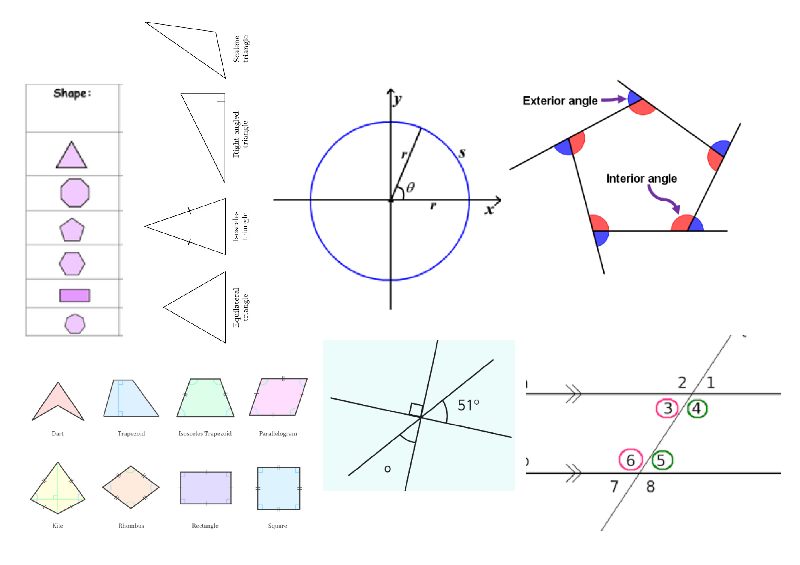Angles at a Point MANIPULATIVE
An angle is measured with reference to a circle with its centre at the common endpoint of the rays. Hence, the angle sum of a circle is 360 degrees.
A5Faae7D6F4867Fd9D6600489Fd60Fb9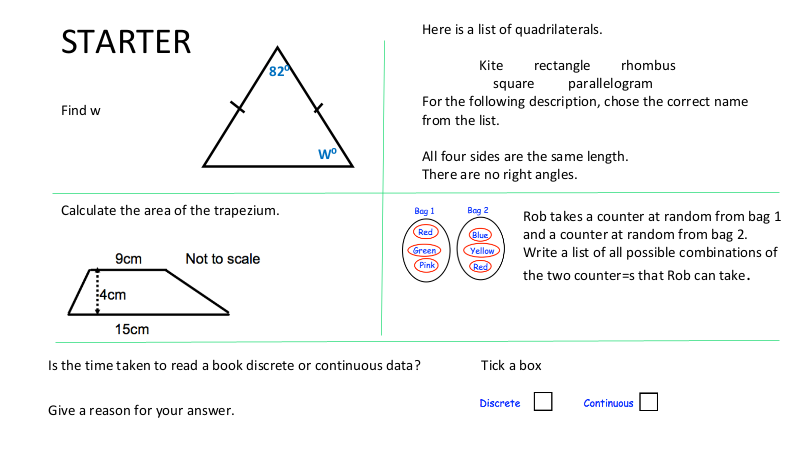Angles on parallel lines VISUAL DEMO
When two lines are crossed by another line (called the Transversal), the angles in matching corners are called Corresponding Angles.